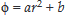# The electrostatic potential inside a charged spherical ball is given by  where r is the distance from the centre; a, b are constants. Then t...

The electrostatic potential inside a charged spherical ball is given bywhere r is the distance from the centre; a, b are constants. Then the charge density inside the ball is

Anonymous User Physics Electrostatic 15 May, 2020 56 views